Related Articles

# Relationship between grammar and Language

• Last Updated : 05 Jul, 2021

Prerequisite – Regular Grammar, Regular Expressions, Chomsky hierarchy

Overview :
In this article, we will discuss the overview of Regular Grammar can be either Right Linear or Left Linear, and mainly focus will be on the relationship between grammar and Language. Let’s discuss it one by one.

Types :
There are two types of grammar as follows.

Right Linear –

``` V->T* + T*V ,
where V - is the variable and T is the set of terminals.```

Left Linear –

``` V -> T* + VT* ,
where V - is the variable and T is the set of terminals.```

Relationship between grammar and Language :
There are some model grammars. By using them, we can learn to derive regular grammar for different Regular Languages, Regular expressions.

Example –
Let r be the regular expression and for each regular expression, regular grammar corresponds to the particular regular expression is given below.

Case-1 :
When r = Φ, at least we should have one production.
Regular grammar –

`S→A`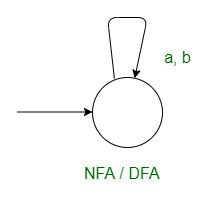Case-2 :
When r = ε,
Regular grammar –

`S→ε`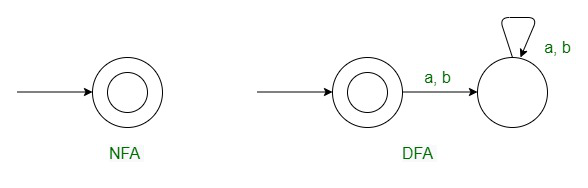Case-3 :
When r=a,
Regular grammar –

`S→a`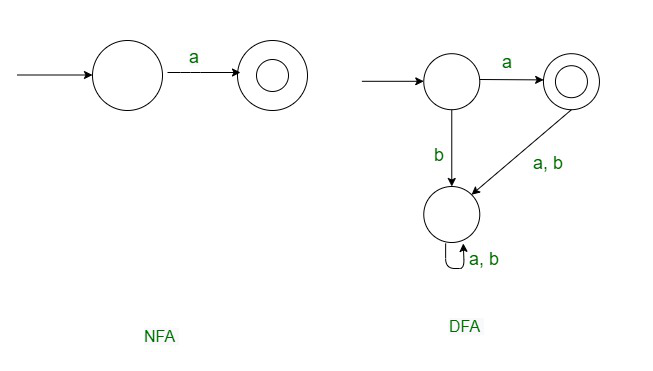Case-4 :
When r=a+b,
Regular grammar –

`S→ a | b`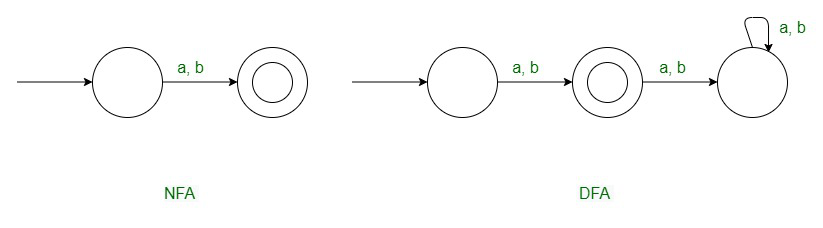Case-5 :
When r = a
Right Linear Grammar –

`S→aS | ε `

Left Linear Grammar –

`S→Sa | ε`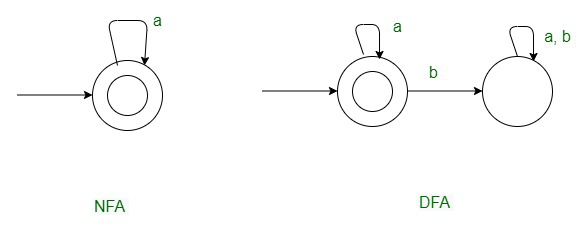Case-6 :
When r= X*
Regular grammar –

`S→XS | ε `

Case-7 :
When r=X×y
Right Linear Grammar –

` S→XS | y `

Case-8 :
r= a*+b*
Regular grammar –

```     S → S1 | S2
S1 → aS1 | ε
S2 → bS2 | ε```

Case-9 :
When r= a*. b*
Right linear –

```    S → aS | S1
S1 → bS1 | ε```

Left linear –

```   S → Sb | S1
S1 → Sa | ε ```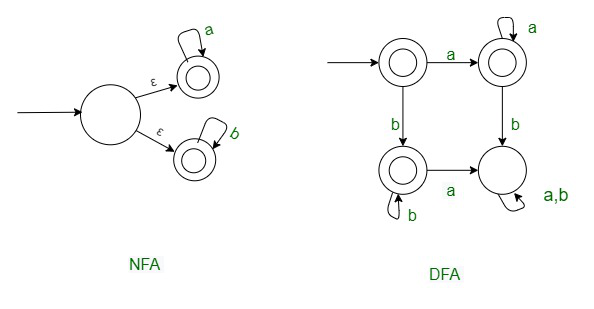Case-10 :
r = (a+b)*

Right linear –

`S→ aS | bS | ε`

Left linear –

`S→ Sa | Sb | ε`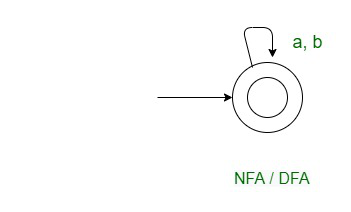Case-11 :
r = (a+b)+

Right linear –

`S→ a | b | aS | bS`

Left linear –

`S→ a | b | Sa | Sb`

Case-12 :
r = X*zY*

`Regular grammar : S→ XS | Sy | z `

Case-13 :
r= (X+Y)*z
Regular grammar :

```S→ XS | YS | z
S → SY | SX | z```

Attention reader! Don’t stop learning now.  Practice GATE exam well before the actual exam with the subject-wise and overall quizzes available in GATE Test Series Course.

Learn all GATE CS concepts with Free Live Classes on our youtube channel.

My Personal Notes arrow_drop_up